HETEROSKEDASTIC ORDERED PROBIT MODELS

Ordinal variables are categorical and ordered, such as poor, fair, good, very good, and excellent. One way to think about ordered variables is that the categories represent ranges of an unobserved continuous variable z. The mapping might be
```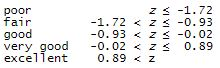```

Perhaps the categories are for overall health.

If z were distributed normal with mean 0 and standard deviation 1, the above would be an ordered probit model. It would correspond to 4% of subjects reporting poor, 13% reporting fair, and so on.

Stata would fit this model if you used its ordered probit command oprobit and typed

```. oprobit health

```

You could instead specify a linear function for z in terms of agebmi, and i.exercise by typing

```. oprobit health age bmi i.exercise

```

The fitted model might be

```z = -0.0083*age - 0.0469*bmi + 0.5596*i.exercise

and the corresponding mapping be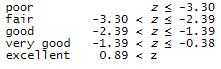```

It would, however, be reasonable to assume that health status varies more as age increases; Stata’s new hetoprobit command can handle that. You would type

```. hetoprobit health age bmi i.exercise, het(age)

```

Let’s see it work

We have data from the 2015 Eating & Health Module of the American Time Use Survey (ATUS) of the U.S. Bureau of Labor Statistics. If we type the above command with these data, the result is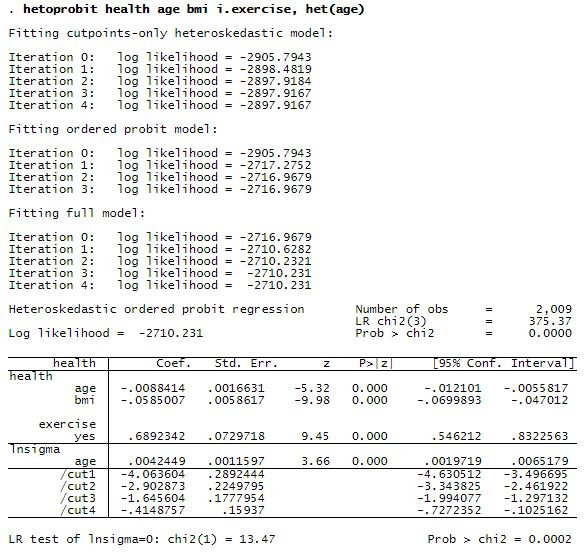The result is

```z = -0.0088*age - 0.0585*bmi + 0.6892*i.exercise

```

with corresponding mapping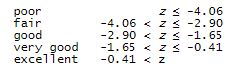These results account for the possible increased variation (heteroskedasticity) due to age.

Is there such heteroskedasticity? There is according to the output chi2(1) = 13.47.

Does it make a difference? Let’s compare coefficients with those we previously calculated using oprobit:

```z = -0.0083*age - 0.0469*bmi + 0.5596*i.exercise  (orig.)
z = -0.0088*age - 0.0585*bmi + 0.6892*i.exercise  (adj)
```

The effect of i.exercise has changed, although perhaps not significantly.

Here is a graph of the predicted probability of very good or excellent health against body mass index.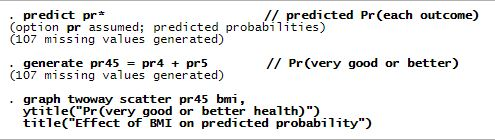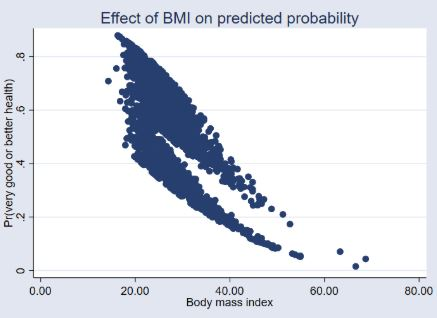Does the division into distinct groups surprise you? It did us. It turns out that the top group exercises and the lower group does not.